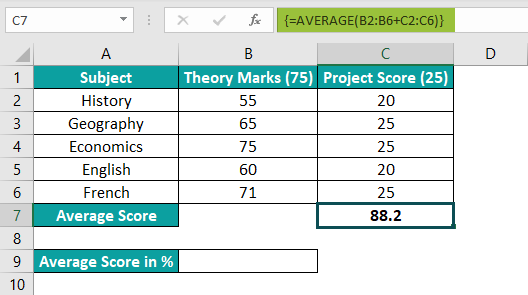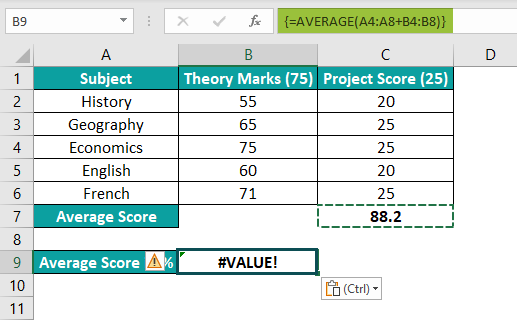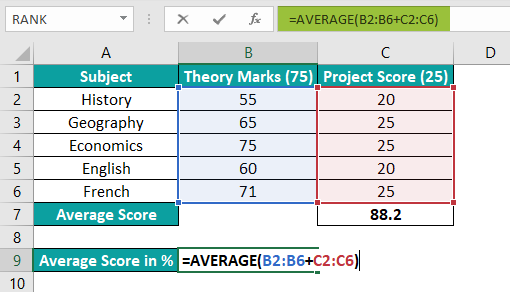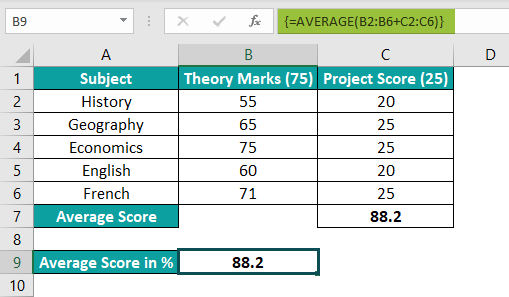# Array Formulas In ExcelArticle byExcelMojo Team## What Is Array Formula In Excel?

The Array Formula in Excel performs multiple calculations on the dataset values of rows, columns, or a cell range. It returns single or multiple results as required in the formula.

One Array Formula in Excel replaces multiple formulas that enable users to apply standard Excel functions to several cells quickly. And all formulas in the selected array range will be the same, so they ensure consistency. For example, the following table shows the annual salary and increment details from 2015 to 2020.

We will calculate the total earnings during the six years and display the output in cells D2:D7 using the Array Formula in Excel. Select cells D2:D7, enter the formula =B2:B7+C2:C7, and press the keys Ctrl+Shift+Enter, the final formula will automatically change to {=B2:B7+C2:C7} within curly brackets.

The output is shown above in Column D, as we had selected cell range D2:D7.

###### Key Takeaways
• Array Formulas in Excel perform several calculations on values in a single array or multiple arrays at once, thus reducing the evaluation time and complexity.
• We can use Array Formulas for functions such as SUM and AVERAGE that return a single cell output and for functions like TRANSPOSE and LINEST that return the result in a single array or multiple arrays.
• We must always press the shortcut keys Ctrl+Shift+Enter to execute the formula as an array function. If we press the Enter key, we will get the result only for the first data.

### How To Enter An Array Formula? (CTRL+SHIFT+ENTER)

We must Enter an Array Formula as follows:

• First, select the cell or cell range for the output.
• Next, type the cell range or select the range from the dataset.
• Enter the right arithmetic operators as +, -, *, /, for their respective types of calculations.
• Finally, press the keys Ctrl+Shift+Enter [a.k.a the CSE formula].

[Special Note: Few points to keep in mind while entering the shortcut keys Ctrl+Shift+Enter, to enable Array Formulas in Excel are:

• When we enter the formula and press the shortcut keys, we can see in the Formula Bar that the formula appears within the curly brackets, indicating that the function is now an Array Formula.
• Every time we edit the Array Formula, press Ctrl+Shift+Enter, to execute it.
• Only the curly braces that appear will enable Array Formulas in Excel.]

### How To Use Array Formula in Excel?

We can use Array Formula in Excel in two ways:

• Single-cell Formula – The input can be a single array or multiple arrays, but the result will be a single-cell output since one cell is selected for the output.
• Multi-cell Formula – The input can be a single array or multiple arrays, but the output is displayed in the selected cell range.

#Basic Example

The following example will help us in understanding Array Formulas in Excel, with the monthly income and expense. We will find the total savings made during the year.

In the table, the data is,

• Column A contains the Months.
• Column B contains the Monthly Income.
• Column C contains the Monthly Expenses.

The steps to find the total savings made during the year using the Array Formula in Excel are:

Step 1: Select cell B15 and enter the formula =SUM(B2:B13-C2:C13).

Step 2: Press the shortcut keys Ctrl+Shift+Enter to execute the Array Formula.

The output is shown above. The final formula appears within curly brackets in the Formula Bar.

### Examples

Let us understand Array Formulas in Excel with some advanced scenarios.

#### #Example 1

The following illustration explains a single cell output. We will compare steps to find the output using the Standard Excel and an Array Function. We will find the lowest difference in units sold between the first half and second half of a year,

In the following table, the data is,

• Column A contains the Smartphones.
• Column B contains the Jan to Jun Units Sold.
• Column C contains the Jul to Dec Units Sold.
• Columns D, H, and I for the outputs.

The steps to find the lowest difference using the Standard Excel function are:

1: Choose cell D2, enter the formula =C2-B2, and press the “Enter” key. [This will determine the difference in the units sold between the two durations for the first smartphone in cell A2].

2: Drag the formula using the fill handle from cell D2 to D10.

3: Next, choose cell G2 to enter the MIN() formula =MIN(D2:D10) and press the “Enter” key. We will get the output as shown in the image below.

4: Now, to determine the smartphone model with the least units sold difference, choose cell H2 and enter the formula =INDEX(A2:A10,MATCH(MIN(D2:D10),D2:D10,FALSE),1)

We will get the output as shown above. [i.e., The smartphone model that has the lowest difference in units sold between the first half and second half of a year].

Now, let us use the other method.

The steps to find the lowest difference using the Array Formulas in Excel are:

Now, when we use Array Formulas in Excel, the steps will be:

Step 1: Choose cell G3 and enter the formula =MIN(C2:C10-B2:B10).

Step 2: Press the shortcut keys Ctrl+Shift+Enter to complete the action.

The two arrays, B2:B10 and C2:C10, are the input. And the expression {=MIN(C2:C10-B2:B10)} first calculates the unit sold difference for each smartphone model and then determines the least value among them i.e., -1952.

Step 3: Drag the formula using the fill handle in cell H2 to H3, or we can copy the formula. The output will be as shown in the image below.

Note the Observation:

• When applied as a standard Excel function, the MIN() takes three tedious steps to determine the required value.
• When we use it as an Array Formula, we only enter the function in the target cell G3 directly instead of calculating the values of column D. Within two steps, we get the result].

#### #Example 2

We will now see how the Array Formulas in Excel work when the output is for multiple cells.

In the following table, the data is,

• Column A contains the Student Names.
• Column B contains the English Scores.
• Column C contains the Credits Out of 20.
• Column D contains the Overall English Score.

The steps to determine the overall English score for each using the Array Formula in Excel are:

Step 1: Select the cell range D2:D11 and enter the formula =B2:B11+C2:C1.

Step 2: Press the Array Formulas in Excel shortcut keys Ctrl+Shift+Enter to execute the formula.

The output is shown above. The Excel array function, {=B2:B11+C2:C11}, takes two arrays, B2:B11, and C2:C11, as the input. It then adds the English score and credits for each student and displays the individual total scores for the ten students in the cell range D2:D11 i.e., multiple cell range output.

#### #Example 3

One of the best examples of Array Formulas in Excel with multiple arrays as input and output is using the TRANSPOSE() function for the following population data.

In the table below, the data is,

• Column A contains the Country.
• Column B contains the Rank.
• Column C contains the Population.

The steps to use the Array Formulas in Excel and the Transpose() function are as follows:

1: Choose cell range A9:F11 to display the transposed table. [Note: The target table’s rows-columns cell counts should match the source table’s columns-rows cell counts, i.e., here source table is (3 columns * 6 rows) = 18 cells, so selected range for target table is (3 rows * 6 columns) = 18 cells].

2: Now, enter the formula =TRANSPOSE(A1:C6).

3: Press the shortcut keys, Ctrl+Shift+Enter, to execute the function as an Array Formula. The output is shown in the image below.

When using multi-cell array functions, we must remember the below points while updating Array Formulas in Excel.

• Choose the target cell range where we want to display the output and then enter the formula.
• The selected target cell range should have the correct size. Otherwise, a smaller selection will not display the entire output, while a larger selection will return an #N/A error.
• To remove a multi-cell array function, choose the entire cell range where we applied the formula and press Delete. Alternatively, we can select the formula in the Formula Bar, press Delete, and then press the keys Ctrl+Shift+Enter together.
• If we want to update the formula to apply it to fewer target cells, delete the command and enter the new function with the required cell range.
• While updating Array Formulas in Excel to apply them to new cells, select all the existing and the new cells and enter into the edit mode. Then type in the command with the updated cell range and press the Excel array function keyboard shortcut to apply the updated array function.
• The multiple arrays output scenario does not work when using Excel tables. Also, we cannot edit the data in the cells referenced in the multi-cell Array Formula.

### Using F9 To Evaluate Array Formula

We will use the function key F9 To Evaluate Array Formula for the total expenses incurred from the seafood purchase.

In the following table, the data is,

• Column A contains the Seafood.
• Column B contains the Units Bought.
• Column C contains the Price Per Unit.

Also, the two arrays, B2:B7 and C2:C7, are the input. The formula {=SUM(B2:B7*C2:C7)} in cell E2 first calculates the total amount spent on each item and then adds all the values to determine the total expenses, 927.

The steps to understand the internal evaluation of the above calculation using the F9 key are:

Step 1: Choose the required arguments we want to evaluate in the array function i.e., in the Formula Bar, select cell E2 formula arguments B2:B7*C2:C7.

Step 2: Now, press the F9 key to see how the arguments evaluate to give the final output.

The Formula Bar shows how the chosen terms calculate the product of units bought and the respective price per unit for each item. The SUM() Excel function then adds these products to determine the final output.

[Note: Ensure we select the part of the array function we want to evaluate and press F9. Otherwise, if we place the cursor on the formula and press F9, the key will replace the formula with the value we determined. To exit the Array Formulas in Excel evaluation process, we need to press the Esc key].

### Important Things To Note

• Array Formulas in Excel help execute multiple calculations and process values in a single cell or multiple cells.
• Select the correct target cell range when the output is in multiple arrays to avoid incorrect results.
• If we want to edit an Array Formula,
1. Delete the function and re-enter it.
2. Update the new cell references in the function in the edit mode and press Ctrl+Shift+Enter to execute the command.
3. If we get a #Value! error, so go again and press Ctrl+Shift+Enter to execute and exit.

How to copy and paste Array Formulas in Excel?

We can copy and paste Array Formulas in Excel by copying the function from the source cell or cell range and pasting it into the chosen cell or cell range. We then must update the precise cell range references and press Ctrl+Shift+Enter to execute the Array Formula in the target cell or cells.

For example, the table below contains a student’s Subject scores, i.e., Theory Marks and Project Scores.The steps to copy and paste the Array Formula from cell C7 to cell B9 are:

1: Copy the Array Formula from cell C7 and paste it into the target cell B9. [Note: We can ignore the #Value! Error“].2: Update the cell range references in the copied formula in cell B9 i.e., the formula is =AVERAGE(A4:A8+B4:B8) is updated to =AVERAGE(B2:B6+C2:C6)3: Finally, press Ctrl+Shift+Enter. The output is shown below.How to automatically update Array Formulas in Excel?

We can automatically update Array Formulas in Excel using the below steps:

1. If it’s a single-cell Array Formula, choose the cell and press F2 to go to edit mode. Then update the required changes, and press Ctrl+Shift+Enter to execute the updated array function.
2. If it’s a multi-cell Array Formula, and we need to apply it on fewer cells, first delete the formula and enter a new function with the required cell range. Then press Ctrl+Shift+Enter to execute the updated array function.
3. If it’s a multi-cell Array Formula and we need to update it in new cells, select all the existing and the new cells and go to the edit mode. Then type in the formula with the updated cell range and press the Excel array function keyboard shortcut to apply the updated array function.

How to remove Array Formulas in Excel?

We can remove Array Formulas in Excel by choosing the specific cell or the cell range and press the Delete key on the keyboard.